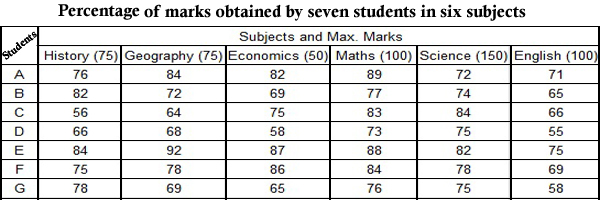## IBPS RRB Quant Test 7

Instructions

Study the following table carefully and answer the questions given below it.Q 1

What is the percentage of marks (rounded-off to two decimals) obtained by student ‘E’ in all subjects together ?

Q 2

What are the average marks obtained (rounded-off to two decimals) by all the students is in Science ?

Q 3

What are the total marks obtained by student ‘C’ in all the subjects together ?

Q 4

What are the total marks obtained by student ‘B’ in History and Economics together ?

Q 5

What are the average marks (rounded-off to two decimals) obtained by all the students in Economics ?